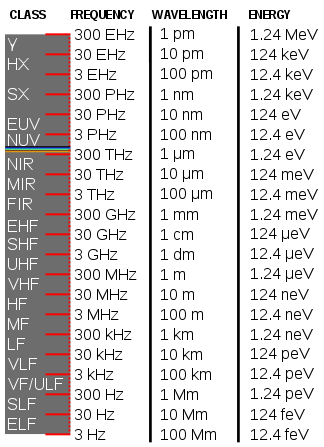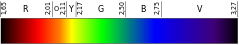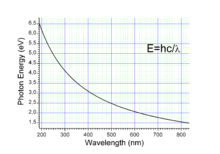# Electronvolt

meV, keV, MeV, GeV, TeV and PeV redirect here. For other uses, see MEV, KEV, GEV, TEV and PEV.

In physics, the electronvolt (symbol eV, also written electron volt) is a unit of energy equal to approximately 160 zeptojoules (10−21 joules, symbol zJ) or 1.6×10−19 joules (symbol J). By definition, it is the amount of energy gained (or lost) by the charge of a single electron moving across an electric potential difference of one volt. Thus it is 1 volt (1 joule per coulomb, 1 J/C) multiplied by the elementary charge (e, or 1.6021766208(98)×10−19 C). Therefore, one electronvolt is equal to 1.6021766208(98)×10−19 J. Historically, the electronvolt was devised as a standard unit of measure through its usefulness in electrostatic particle accelerator sciences because a particle with charge q has an energy E = qV after passing through the potential V; if q is quoted in integer units of the elementary charge and the terminal bias in volts, one gets an energy in eV.The electronvolt is not an SI unit, and its definition is empirical (unlike the litre, the light-year and other such non-SI units), thus its value in SI units must be obtained experimentally. Like the elementary charge on which it is based, it is not an independent quantity but is equal to 1 J/C2hα / μ0c0. It is a common unit of energy within physics, widely used in solid state, atomic, nuclear, and particle physics. It is commonly used with the metric prefixes milli-, kilo-, mega-, giga-, tera-, peta- or exa- (meV, keV, MeV, GeV, TeV, PeV and EeV respectively). Thus meV stands for milli-electronvolt.

In some older documents, and in the name Bevatron, the symbol BeV is used, which stands for billion electronvolts; it is equivalent to the GeV.

Measurement Unit SI value of unit
Energy eV 1.602176565(35)×10−19 J
Mass eV/c2 1.782662×10−36 kg
Momentum eV/c 5.344286×10−28 kg⋅m/s
Temperature eV/kB 1.1604505(20)×104 K
Time ħ/eV 6.582119×10−16 s
Distance ħc/eV 1.97327×10−7 m

## Mass

By mass–energy equivalence, the electronvolt is also a unit of mass. It is common in particle physics, where units of mass and energy are often interchanged, to express mass in units of eV/c2, where c is the speed of light in vacuum (from E = mc2). It is common to simply express mass in terms of "eV" as a unit of mass, effectively using a system of natural units with c set to 1. The mass equivalent of 1 eV/c2 isFor example, an electron and a positron, each with a mass of 0.511 MeV/c2, can annihilate to yield 1.022 MeV of energy. The proton has a mass of 0.938 GeV/c2. In general, the masses of all hadrons are of the order of 1 GeV/c2, which makes the GeV (gigaelectronvolt) a convenient unit of mass for particle physics:

1 GeV/c2 = 1.783×10−27 kg.

The unified atomic mass unit (u), 1 gram divided by Avogadro's number, is almost the mass of a hydrogen atom, which is mostly the mass of the proton. To convert to megaelectronvolts, use the formula:

1 u = 931.4941 MeV/c2 = 0.9314941 GeV/c2.

## Momentum

In high-energy physics, the electronvolt is often used as a unit of momentum. A potential difference of 1 volt causes an electron to gain an amount of energy (i.e., 1 eV). This gives rise to usage of eV (and keV, MeV, GeV or TeV) as units of momentum, for the energy supplied results in acceleration of the particle.

The dimensions of momentum units are LMT−1. The dimensions of energy units are L2MT−2. Then, dividing the units of energy (such as eV) by a fundamental constant that has units of velocity (LT−1), facilitates the required conversion of using energy units to describe momentum. In the field of high-energy particle physics, the fundamental velocity unit is the speed of light in vacuum c. Thus, dividing energy in eV by the speed of light, one can describe the momentum of an electron in units of eV/c. 

The fundamental velocity constant c is often dropped from the units of momentum by way of defining units of length such that the value of c is unity. For example, if the momentum p of an electron is said to be 1 GeV, then the conversion to MKS can be achieved by:## Distance

In particle physics, a system of "natural units" in which the speed of light in vacuum c and the reduced Planck constant ħ are dimensionless and equal to unity is widely used: c = ħ = 1. In these units, both distances and times are expressed in inverse energy units (while energy and mass are expressed in the same units, see mass–energy equivalence). In particular, particle scattering lengths are often presented in units of inverse particle masses.

Outside this system of units, the conversion factors between electronvolt, second, and nanometer are the following:The above relations also allow expressing the mean lifetime τ of an unstable particle (in seconds) in terms of its decay width Γ (in eV) via Γ = ħ/τ. For example, the B0 meson has a lifetime of 1.530(9) picoseconds, mean decay length is = 459.7 µm, or a decay width of (4.302±25)×10−4 eV.

Conversely, the tiny meson mass differences responsible for meson oscillations are often expressed in the more convenient inverse picoseconds.

## Temperature

In certain fields, such as plasma physics, it is convenient to use the electronvolt as a unit of temperature. The conversion to the Kelvin scale is defined by using kB, the Boltzmann constant:For example, a typical magnetic confinement fusion plasma is 15 keV, or 170 megakelvin.

As an approximation: kBT is about 0.025 eV (≈ 290 K/11604 K/eV) at a temperature of 20 °C.

## PropertiesEnergy of photons in the visible spectrum in eVGraph of wavelength (nm) to energy (eV)

The energy E, frequency v, and wavelength λ of a photon are related bywhere h is the Planck constant, c is the speed of light. This reduces toA photon with a wavelength of 532 nm (green light) would have an energy of approximately 2.33 eV. Similarly, 1 eV would correspond to an infrared photon of wavelength 1240 nm or frequency 241.8 THz.

## Scattering experiments

In a low-energy nuclear scattering experiment, it is conventional to refer to the nuclear recoil energy in units of eVr, keVr, etc. This distinguishes the nuclear recoil energy from the "electron equivalent" recoil energy (eVee, keVee, etc.) measured by scintillation light. For example, the yield of a phototube is measured in phe/keVee (photoelectrons per keV electron-equivalent energy). The relationship between eV, eVr, and eVee depends on the medium the scattering takes place in, and must be established empirically for each material.

## Energy comparisons

• 5.25×1032 eV: total energy released from a 20 kt nuclear fission device
• 1.22×1028 eV: the Planck energy
• 1×1025 eV: the approximate grand unification energy
• ~624 EeV (6.24×1020 eV): energy consumed by a single 100-watt light bulb in one second (100 W = 100 J/s6.24×1020 eV/s)
• 300 EeV (3×1020 eV = ~50 J): the so-called Oh-My-God particle (the most energetic cosmic ray particle ever observed)
• 2 PeV: two petaelectronvolts, the most high-energetic neutrino detected by the IceCube neutrino telescope in Antarctica
• 14 TeV: the designed proton collision energy at the Large Hadron Collider (operated at about half of this energy since 30 March 2010, reached 13TeV in May 2015)
• 1 TeV: a trillion electronvolts, or 1.602×10−7 J, about the kinetic energy of a flying mosquito
• 125.1±0.2 GeV: the energy corresponding to the mass of the Higgs boson, as measured by two separate detectors at the LHC to a certainty better than 5 sigma
• 210 MeV: the average energy released in fission of one Pu-239 atom
• 200 MeV: the average energy released in nuclear fission of one U-235 atom
• 17.6 MeV: the average energy released in the fusion of deuterium and tritium to form He-4; this is 0.41 PJ per kilogram of product produced
• 1 MeV (1.602×10−13 J): about twice the rest energy of an electron
• 13.6 eV: the energy required to ionize atomic hydrogen; molecular bond energies are on the order of 1 eV to 10 eV per bond
• 1.6 eV to 3.4 eV: the photon energy of visible light

### Per mole

One mole of particles given 1 eV of energy has approximately 96.5 kJ of energy – this corresponds to the Faraday constant (F 96485 C mol−1) where the energy in joules of N moles of particles each with energy X eV is X·F·N.

## Notes and references

1. IUPAC Gold Book, p. 75
2. SI brochure, Sec. 4.1 Table 7 Archived July 16, 2012, at the Wayback Machine.
3. "CODATA Value: elementary charge". The NIST Reference on Constants, Units, and Uncertainty. US National Institute of Standards and Technology. June 2015. Retrieved 2015-09-22.
4. "CODATA Value: electron volt". The NIST Reference on Constants, Units, and Uncertainty. US National Institute of Standards and Technology. June 2015. Retrieved 2015-09-22.
5. What is Light?UC Davis lecture slides
6. Elert, Glenn. "Electromagnetic Spectrum, The Physics Hypertextbook". hypertextbook.com. Retrieved 2016-07-30.
7. "Definition of frequency bands on". Vlf.it. Retrieved 2010-10-16.
8. Barrow, J. D. "Natural Units Before Planck." Quarterly Journal of the Royal Astronomical Society 24 (1983): 24.
9. "Units in particle physics". Associate Teacher Institute Toolkit. Fermilab. 22 March 2002. Retrieved 13 February 2011.
10. "Special Relativity". Virtual Visitor Center. SLAC. 15 June 2009. Retrieved 13 February 2011.
11. "CODATA Value: Planck constant in eV s". Retrieved 30 March 2015.
12. Open Questions in Physics. German Electron-Synchrotron. A Research Centre of the Helmholtz Association. Updated March 2006 by JCB. Original by John Baez.
13. Glossary - CMS Collaboration, CERN
14. ATLAS; CMS (26 March 2015). "Combined Measurement of the Higgs Boson Mass in pp Collisions at √s=7 and 8 TeV with the ATLAS and CMS Experiments". Physical Review Letters. 114 (19): 191803. arXiv:1503.07589. Bibcode:2015PhRvL.114s1803A. doi:10.1103/PhysRevLett.114.191803. PMID 26024162.